Anzeige

4. May 2015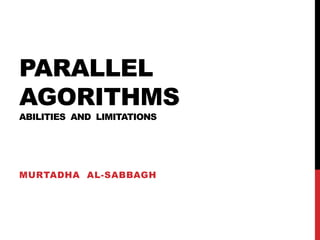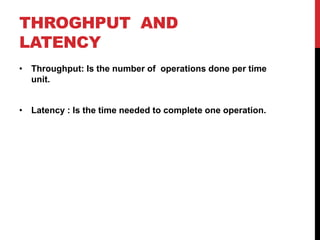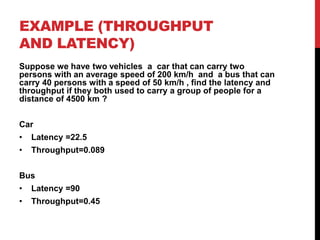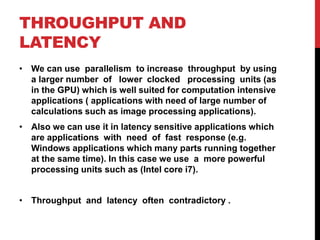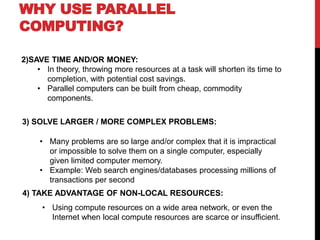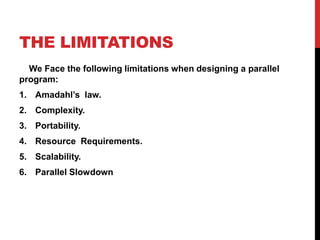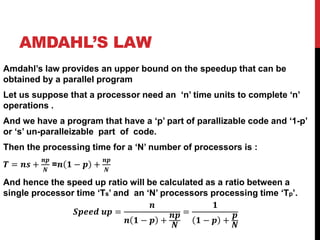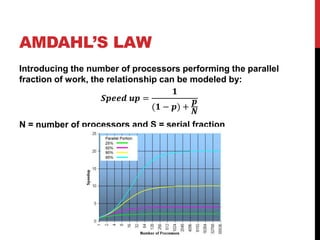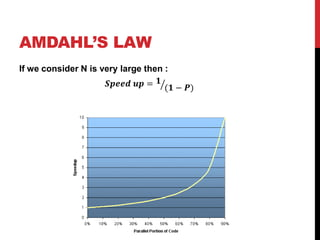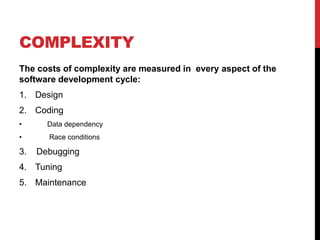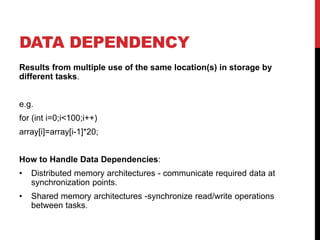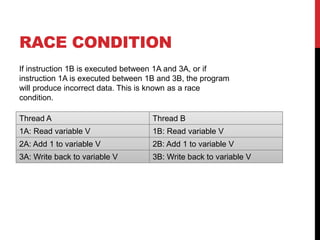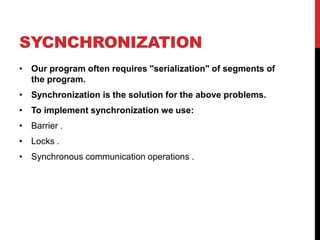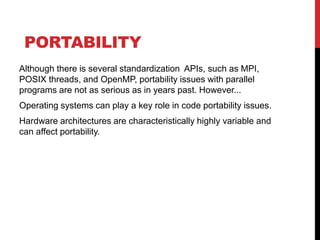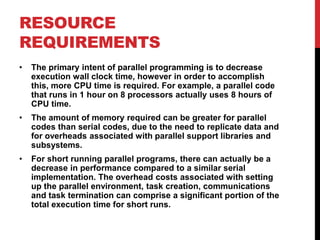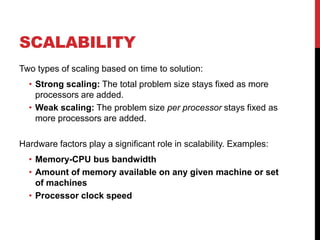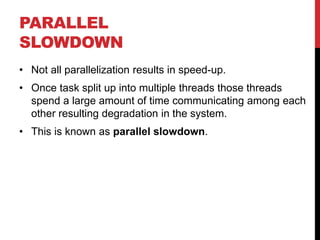1 von 22
Anzeige

1. PARALLEL AGORITHMS ABILITIES AND LIMITATIONS MURTADHA AL-SABBAGH
2. PARALLEL ALGORITHMS ABILITES
3. THROGHPUT AND LATENCY • Throughput: Is the number of operations done per time unit. • Latency : Is the time needed to complete one operation.
4. EXAMPLE (THROUGHPUT AND LATENCY) Suppose we have two vehicles a car that can carry two persons with an average speed of 200 km/h and a bus that can carry 40 persons with a speed of 50 km/h , find the latency and throughput if they both used to carry a group of people for a distance of 4500 km ? Car • Latency =22.5 • Throughput=0.089 Bus • Latency =90 • Throughput=0.45
5. THROUGHPUT AND LATENCY • We can use parallelism to increase throughput by using a larger number of lower clocked processing units (as in the GPU) which is well suited for computation intensive applications ( applications with need of large number of calculations such as image processing applications). • Also we can use it in latency sensitive applications which are applications with need of fast response (e.g. Windows applications which many parts running together at the same time). In this case we use a more powerful processing units such as (Intel core i7). • Throughput and latency often contradictory .
6. WHY USE PARALLEL COMPUTING? 1) The Real World is Massively Parallel: In the natural world, many complex, interrelated events are happening at the same time, yet within a temporal sequence. Compared to serial computing, parallel computing is much better suited for modeling, simulating and understanding complex, real world phenomena.
7. For example, imagine modeling these serially:
8. WHY USE PARALLEL COMPUTING? 2)SAVE TIME AND/OR MONEY: • In theory, throwing more resources at a task will shorten its time to completion, with potential cost savings. • Parallel computers can be built from cheap, commodity components. 3) SOLVE LARGER / MORE COMPLEX PROBLEMS: • Many problems are so large and/or complex that it is impractical or impossible to solve them on a single computer, especially given limited computer memory. • Example: Web search engines/databases processing millions of transactions per second • Using compute resources on a wide area network, or even the Internet when local compute resources are scarce or insufficient. 4) TAKE ADVANTAGE OF NON-LOCAL RESOURCES:
9. PARALLEL ALGORITHMS LIMITS
10. THE LIMITATIONS We Face the following limitations when designing a parallel program: 1. Amadahl’s law. 2. Complexity. 3. Portability. 4. Resource Requirements. 5. Scalability. 6. Parallel Slowdown
11. AMDAHL’S LAW Amdahl’s law provides an upper bound on the speedup that can be obtained by a parallel program Let us suppose that a processor need an ‘n’ time units to complete ‘n’ operations . And we have a program that have a ‘p’ part of parallizable code and ‘1-p’ or ‘s’ un-paralleizable part of code. Then the processing time for a ‘N’ number of processors is : 𝑻 = 𝒏𝒔 + 𝒏𝒑 𝑵 =𝒏 𝟏 − 𝒑 + 𝒏𝒑 𝑵 And hence the speed up ratio will be calculated as a ratio between a single processor time ‘Ts’ and an ‘N’ processors processing time ‘Tp’. 𝑺𝒑𝒆𝒆𝒅 𝒖𝒑 = 𝒏 𝒏 𝟏 − 𝒑 + 𝒏𝒑 𝑵 = 𝟏 𝟏 − 𝒑 + 𝒑 𝑵
12. AMDAHL’S LAW Introducing the number of processors performing the parallel fraction of work, the relationship can be modeled by: 𝑺𝒑𝒆𝒆𝒅 𝒖𝒑 = 𝟏 (𝟏 − 𝒑) + 𝒑 𝑵 N = number of processors and S = serial fraction
13. AMDAHL’S LAW If we consider N is very large then : 𝑺𝒑𝒆𝒆𝒅 𝒖𝒑 = 𝟏 (𝟏 − 𝑷)
14. COMPLEXITY The costs of complexity are measured in every aspect of the software development cycle: 1. Design 2. Coding • Data dependency • Race conditions 3. Debugging 4. Tuning 5. Maintenance
15. DATA DEPENDENCY Results from multiple use of the same location(s) in storage by different tasks. e.g. for (int i=0;i<100;i++) array[i]=array[i-1]*20; How to Handle Data Dependencies: • Distributed memory architectures - communicate required data at synchronization points. • Shared memory architectures -synchronize read/write operations between tasks.
16. RACE CONDITION Thread A Thread B 1A: Read variable V 1B: Read variable V 2A: Add 1 to variable V 2B: Add 1 to variable V 3A: Write back to variable V 3B: Write back to variable V If instruction 1B is executed between 1A and 3A, or if instruction 1A is executed between 1B and 3B, the program will produce incorrect data. This is known as a race condition.
17. SYCNCHRONIZATION • Our program often requires "serialization" of segments of the program. • Synchronization is the solution for the above problems. • To implement synchronization we use: • Barrier . • Locks . • Synchronous communication operations .
18. PORTABILITY Although there is several standardization APIs, such as MPI, POSIX threads, and OpenMP, portability issues with parallel programs are not as serious as in years past. However... Operating systems can play a key role in code portability issues. Hardware architectures are characteristically highly variable and can affect portability.
19. RESOURCE REQUIREMENTS • The primary intent of parallel programming is to decrease execution wall clock time, however in order to accomplish this, more CPU time is required. For example, a parallel code that runs in 1 hour on 8 processors actually uses 8 hours of CPU time. • The amount of memory required can be greater for parallel codes than serial codes, due to the need to replicate data and for overheads associated with parallel support libraries and subsystems. • For short running parallel programs, there can actually be a decrease in performance compared to a similar serial implementation. The overhead costs associated with setting up the parallel environment, task creation, communications and task termination can comprise a significant portion of the total execution time for short runs.
20. SCALABILITY Two types of scaling based on time to solution: • Strong scaling: The total problem size stays fixed as more processors are added. • Weak scaling: The problem size per processor stays fixed as more processors are added. Hardware factors play a significant role in scalability. Examples: • Memory-CPU bus bandwidth • Amount of memory available on any given machine or set of machines • Processor clock speed
21. PARALLEL SLOWDOWN • Not all parallelization results in speed-up. • Once task split up into multiple threads those threads spend a large amount of time communicating among each other resulting degradation in the system. • This is known as parallel slowdown.
22. THANK YOU ~_^

### Hinweis der Redaktion

1. The Same law in the next page except 8
Anzeige